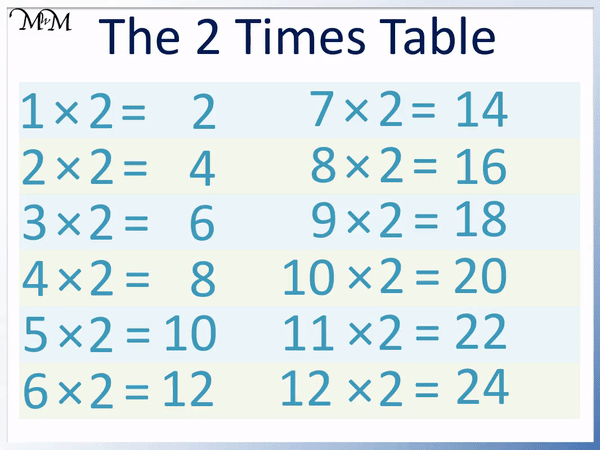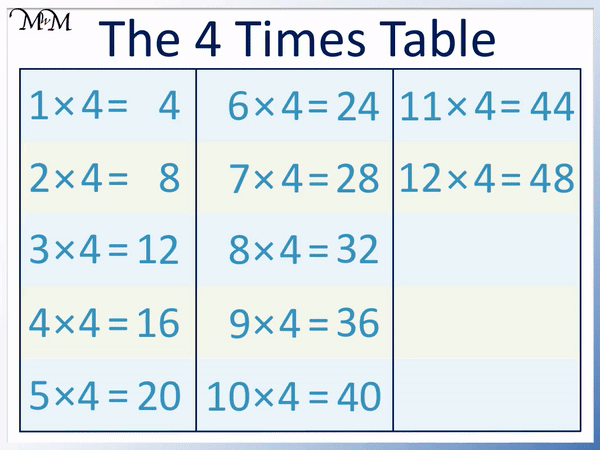# The 4 Times Table

The 4 Times TableThe 4 times table is:

• 1 × 4 = 4
• 2 × 4 = 8.
• 3 × 4 = 12
• 4 × 4 = 16
• 5 × 4 = 20
• 6 × 4 = 24
• 7 × 4 = 28
• 8 × 4 = 32
• 9 × 4 = 36
• 10 × 4 = 40
• 11 × 4 = 44
• 12 × 4 = 48• To learn the four times table, it is helpful to already know the two times table.
• The 4 times table is simply double the 2 times table.
• To double the answers of the 2 times table, we simply add the number to itself.
• For example if we know that 5 × 2 = 10, we can double 10 to see that 5 × 4 = 20.
• To multiply a number by 4, double it and double it again.
• The last digits of the 4 times table repeat the pattern of 4, 8, 2, 6 and 0.

To multiply a number by 4, double it and double it again.• To multiply a number by 3, double the number and double it again.
• To double a number, add it to itself.
• 3 + 3 = 6 and so, doubling 3 equals 6.
• 6 + 6 = 12 and so, doubling 6 equals 12.
• 3 × 4 = 12#### 4 Times Table Flashcards

Click on the 4 times table flashcards below to memorise the 4 times table.

×
×

=

# The 4 Times Table

## What is the 4 Times Table

The 4 times table is:

• 1 × 4 = 4
• 2 × 4 = 8
• 3 × 4 = 12
• 4 × 4 = 16
• 5 × 4 = 20
• 6 × 4 = 24
• 7 × 4 = 28
• 8 × 4 = 32
• 9 × 4 = 36
• 10 × 4 = 40
• 11 × 4 = 44
• 12 × 4 = 48

Below is a chart showing the complete 4 times table.The 4 times table can be formed by counting in fours.

Simply add 4 to any number in the 4 times table to get to the next number in the 4 times table.

## How to Learn the 4 Times Table

The easiest way to learn the 4 times table is to double the answers in the 2 times table. To multiply a number by 4, simply double it and double it again.

To double a number, simply add it to itself.

The 4 times table repeats the pattern of digits ending in 4, 8, 2, 6 and 0. This pattern can help us to work out the next number in the 4 times table.

Below is the 2 times table chart.

We can make the 4 times table chart by doubling each answer in the 2 times table chart.For example, to find 2 × 4, we double two and then double it again.

2 + 2 = 4, so 2 doubled is 4.

We then double 4.

4 + 4 = 8.Therefore 2 × 4 = 8.

If we know the 2 times table, then we can easily find the 4 times table.

By remembering 2 × 2 = 4, we can double it to see that 2 × 4 = 8.

Here is another example of 3 × 4.

We double 3 and then double it again.

3 + 3 = 6 and so, 3 doubled is 6.

We then double 6.

6 + 6 = 12 and so, 6 doubled is 12.Therefore 3 × 4 = 12.

If we remember that 3 × 2 = 6, then we can double the result to see that 3 × 4 = 12.

Here is the example of 5 × 4.

To multiply 5 by 4, we double 5 and then double it again.

5 + 5 = 10 and so, 5 doubled is 10.

10 + 10 = 20 and so, 10 doubled is 20.Therefore 5 × 4 = 20.

If we knew that 5 × 2 = 10, then we can double this result to see that 5 × 4 = 20.

We can also remember the 4 times table by recognising that we add 4 to get from one answer to the next.

To help us add 4, we can remember the pattern of the last digits ending in 4, 8, 2, 6 and then 0.

Because the 4 times table ends in only 4, 8, 2, 6 and 0, all numbers in the 4 times table are even. No numbers in the 4 times table are odd.

This pattern of 4, 8, 2, 6, 0 repeats as seen in the 4 times table chart below.Therefore we can use this repeating pattern to help learn which number comes next.

For example, if we remember that 3 × 4 = 12, then we can use this answer to work out 4 × 4.

12 ends in a 2 and the next digit after 2 in the pattern of 4, 8, 2, 6, 0 is a 6.

12 + 4 = 16 and so, 4 × 4 = 16.

We can simply add on 4 from 3 × 4 to find 4 × 4.

If we remember that 10 × 4 = 40, then we can work out 9 × 4 by subtracting 4 from 40.

We can see that 6 is before 0 in the repeating pattern of 4, 8, 2, 6, 0 and so, 40 – 4 = 36.

If 10 × 4 = 40, then we can work out that 9 × 4 = 36.Now try our lesson on Multiplying by 100 where we learn how to multiply by 100.